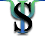# Social Psychology Network

## Maintained by Scott Plous, Wesleyan University

• Directories
• Forums
• MapsRandom Assignment AssignmentA good way to understand random sampling, random assignment, and the difference between the two is to draw a random sample of your own and carry out an example of random assignment. To complete this assignment, begin by opening a second web browser window (or printing this page), and then finish each part in the order below.Part I: Research Randomizer TutorialThe first part of this assignment is to complete a 10-minute web tutorial on random sampling and random assignment. To complete the tutorial:

1. Visit Research Randomizer.

2. Read the introductory page and check that you have an adequate web browser as described under System Requirements (if not, either update your browser or use a different computer).

3. Once you know that your browser is adequate, click on the Tutorial button near the top of the Research Randomizer screen.

4. Go through all four examples in the tutorial.

Tip: After you click on the "Randomize Now!" button to generate numbers in a tutorial lesson, examine the results closely before advancing to the next lesson.Part II: Random Sampling

Suppose you have a numbered list of 2,900 students at your school, and you want to draw a random sample of 60 students for a study you're conducting. In the old days this would have been a time consuming task, but with Research Randomizer, you can generate the results in less than a minute. Here's what you need to do:

1. Click on Randomize near the top of the Research Randomizer screen.

2. Fill in the Randomizer form with the appropriate values.

Tip: There's no need to sort the numbers, because you'll be calling students in the random order that their number appears in your sample.

3. Click the "Randomize Now!" button to get your results.

4. Print out the results to be handed in at the beginning of class next time.Part III: Random Assignment

Suppose you contact the 60 students from Part II to participate in a laboratory study with three conditions, 30 students agree to participate, and you renumber these students from 1 to 30. In this last part of the assignment, your task is to assign each participant to one of three conditions (e.g., so the first person to arrive at the lab is in Condition 2, the next is in Condition 1, the next in Condition 3, the next in Condition 1, the next in Condition 3, the next in Condition 2, and so on).

To complete this step, just generate 10 sets of random numbers ranging from 1 to 3 so that within each block of three students, one person is randomly assigned to each different condition. Here's how:

1. Close the first results window and return to the Randomizer form.

2. Change the Randomizer values as needed.

Tip: Blocked designs are covered in Lesson 3 of the tutorial in Part I above.

3. Click the "Randomize Now!" button to get your new results.

4. Print out the results to be handed in at the beginning of class next time.Return to Top©1996-2021, S. Plous